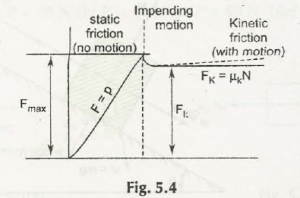In the static frictional force you will get an equation:

F max S N

F max is the maximum static frictional force.

µS is the static frictional coefficient.

In the kinetic frictional force you will get an equation:

Fk= µk N

Here µkis coefficient of kinetic frictional force.

Fk is standing for kinetic frictional force.

Fmax ˃ Fk

µS > µK

Consider this figure for those equations above:Links of Next Mechanical Engineering Topics:-### Customer Reviews

My Homework Help
Rated 5.0 out of 5 based on 510 customer reviews at